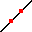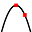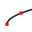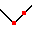Enable text based alternatives for graph display and drawing entry

Try Another Version of This Question

 Describe how the graph of the function y = x+1 can beobtained from one of the basic functions. Then graphthe function at right. Start with the graph of   (enter your basic function as an equation using y= )Then shift the graph unit(s) Select an answer up down to the left to the right Clear All Draw:Describe how the graph of the function y = x^2+2 can beobtained from one of the basic functions. Then graphthe function at right. Start with the graph of   (enter your basic function as an equation, using y= )Then shift the graph unit(s) Select an answer up down to the left to the right . Clear All Draw: